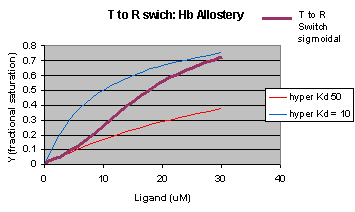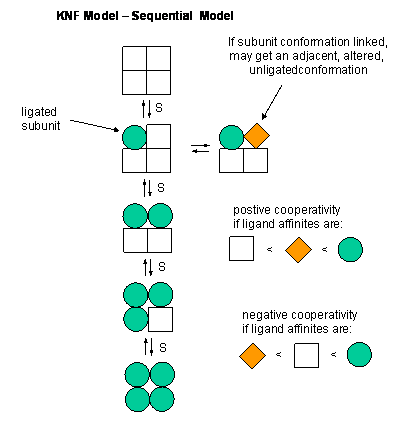# 5.4: Reversible Binding of a Protein to a Ligand - Oxygen-Binding Proteins

## Myoglobin, Hemoglobin, and their Ligands

Almost all biochemistry textbooks start their description of the biological functions of proteins using the myoglobin and hemoglobin as exemplars. These are very rational approaches since they have become model systems to describe the binding of simple ligands, like dioxygen (O2), CO2, and H+, and how the structure of the protein determines and is influenced by binding of ligands.

Yet in a way these "ligands" are dissimilar to perhaps the majority of other proteins which bind small ligands such as substrates (for enzymes), inhibitors and activators or large "ligands" such as other proteins, nucleic acids, carbohydrates and lipids. These type of ligands are reversibly bound through classical intermolecular forces (IMFs), such as hydrogen bonds, London dispersion forces, dipole-dipole interactions, and ion-ion interactions. In addition to these are the less commonly discussed pi-pi (aromatic) interactions and cation-pi (aromatic) interactions.

YouTube: Less Common Discussed IMFs - pi-pi, cation-pi interactions, and halogen bnding

The classic ligands that reversibly bind to hemoglobin, dioxygen, carbon dioxide, and protons, are bound covalently. Dioxygen binds to a heme Fe2+, protons obviously bind to proton acceptors (like His), while CO2 binds covalently as if forms a carbamate with the N terminus of one of the hemoglobin chains.

This discrepancy in ligand binding mode can be explained easily for dioxygen as it forms a coordinate or dative covalent bond with the transition metal ion Fe+2. In ordinary covalent bonds, each bonded atom contributes to and shares the two electron in the bond. In coordinate or dative covalent bonds, the ligand, a Lewis base, contributes both electrons in the bond. Both electrons are still considered to be "owned" by the ligand and not by the transition metal ion, a Lewis acid. Hence the ligand can readily dissociate from the metal ion, much as a ligand bound through classical IMFs can. This analogy can be extended to protons which are also Lewis acids (with no contributing electrons) as they react with Lewis bases (lone pair donors) on atoms such as N on a His side chain.

Mb (a monomer containing 8 α−helices, A-H) and Hb (a heterotetramer with two α -and two β−subunits, each which also contains 8 α−helices) are both oxygen binding proteins. Dioxygen is transported from lungs, gills, or skin of an animal to capillaries, where it can be delivered to respiring tissue. It has a low solubility in blood (0.1 mM). Whole blood, which contains 150 g Hb/L, can carry up to 10 mM dioxygen. Invertebrate can have alternative proteins for oxygen binding, including hemocyanin, which contains Cu and hemerythrin, a non-heme protein. On binding dioxygen, solutions of Hb change color to bright red. Solutions of hemocyanin and hemerythrin change to blue and burgundy colored, respectively, on binding dioxygen. Some Antarctic fish don't require Hb since dioxygen is more soluble at low temperature. Mb is found in the muscle, and serves as a storage protein for oxygen transported by Hb. Some information about the proteins is given below:

Hemoglobin:

• 1st. protein whose molecular weight was determined
• 1st protein to be assigned a specific function - dioxygen transport
• has a prosthetic group (non-amino acid) heme group (protoporphyrin IX with a ferrous ion) covalently attached to the protein. The heme group binds dioxygen.
• 1st protein in which a point mutation (single base pair charge) causes a single amino acid change in the protein, marking the start of molecular medicine
• 1st protein with high resolution x-ray structure
• theory for dioxygen binding explain control of enzyme activity
• the binding of dioxygen is regulated by binding of H+, CO2, and bisphosphoglcyerate which bind to sites (allosteric) distant from oxygen binding site.
• crystals of deoxy-Hb shatter on binding dioxygen, indicating significant conformational changes on binding.

Heme group:

Figure: Heme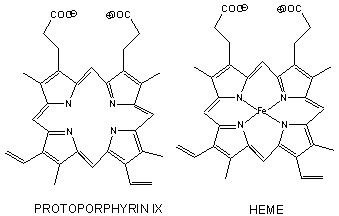• The heme group contains protoporphyrin IX, with four tetrapyrrole rings linked by methene bridges. Attached to the tetrapyrrole structure are four methyl, two vinyl, and two proprionate groups. These can be arranged in 15 ways, only one (IX) occurs in biological systems. Ferrous ion bonded to the protoporphyrin IX constitutes the heme group.
• The heme fits into a hydophobic crevice in the proteins with the proprionate groups exposed to solvent.
• The Fe2+ ion is coordinated to 4 Ns on the 4 pyrrole rings, The 5th ligand is a supplied by proximal His (the 8th amino acid on helix F) of the protein. In the absence of dioxygen, the 6th ligand is missing. and the geometry of the complex is square pyramidal with the Fe above the plane of the heme ring. A distal His (E7) is on the opposite side of the heme ring, but too far to coordinate with the Fe. When dioxgen binds, it occupies the 6th coordination site and pulls the Fe into the plane of the ring, leading to octahedral geometry. CO, NO, and H2S also bind to the 6th site, but with higher affinity than dioxygen, which can lead to CO poisoning. The distal His keeps these ligands (including dioxygen) bound in a bent, non-optimal geometry. This minimizes the chances of CO poisoning.
• When oxygen binds to heme Fe2+, the geometry of the complex becomes octahedral. The Fe2+ has 6 electrons in d orbitals. (The electronic configuration of Fe is 3d64s2 while the Fe2+ ion is 3d6). From crystal field theory (review a general chemistry text), the ligand electrons pairs (treated as anions) interact with the d orbital Fe2+ electrons. They interact most strongly (in a repulsive fashion) with the dz2 and dx2-y2 Fe2+ electrons, which are oriented at the axial ends and equatorial corners, respectively, of the octahedral complex. This repulsion raises the energy of these two orbitals. The other three d orbitals feel less repulsion by the ligand electrons and are lower in energy. The difference in energy between the three lower energy levels of d electrons (in t orbitals) and the higher energy levels of electrons (in g orbitals) allows photons matching that energy difference to be absorbed as electrons are promoted to the g orbitals. Ligands that interact strongly with the dz2 and dx2-y2 electrons raise their energy even more, increasing the energy of the photon required to promote an electron from the t to g orbitals. Dioxygen is one of those ligands, leading to the absorbance of high energy photons, causing the solution to appear bright red (since blue light is absorbed). Because of this strong ligand, the significant energy gap between the t and g orbitals causes the electrons to reside mostly in the lower energy t orbitals where they remained paired, giving the complex a low spin state in which all 6 d electrons are paired. Hence oxyhemoglobin is diagmagnetic. The heme complex can also exist in a high spin state in which the electrons are easily excited to the g orbitals which are closer in energy to the t orbitals when the ligand is weakly interacting with the Fe2+ electrons. In these cases, the heme is paramagnetic since unpaired electrons are possible. (We will discuss paramagnetism latter in the course during our exploration of oxygen chemistry.

Figure: Heme-O2 Octahedral Complex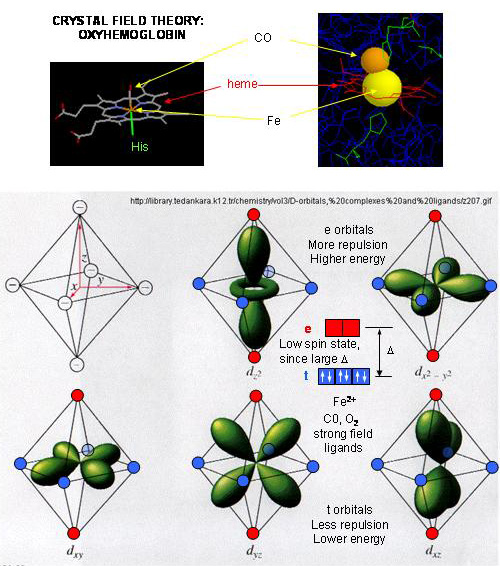• When dioxyen binds, the oxidation state of the Fe2+ ion does not change, even through dioxygen is a great oxidizing agent. Hence the Fe2+ ion is a reversible carrier of dioxygen not of electrons. Free heme in solution is oxidized by dioxygen, forming a complex with water which occupies the 6th position, with the iron in the Fe3+ state. An intermediate in this process is the formation of a dimer of 2 hemes linked by 1 dioxygen. This can't occur readily when the heme is in Hb or Mb. Other heme proteins (like Cytochrome C) are designed to be carriers of electrons.
• A small amount of the Fe2+ ion gets oxidized to Fe3+ ion. Mb and Hb in this state are called met-Hb and met-Mb. A enzyme is required to reduced the iron back to the Fe2+ state.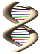Jmol: Updated deoxy-heme and carbon monoxide-heme Jmol14 (Java) | JSMol (HTML5)

Myoglobin

• Mb is extremely compact, and consists of 75% alpha helical structure.
• 8 α−helices are present, labeled A-H.
• 4 alpha helices are terminated by Pro
• The interior amino acids are almost entirely nonpolar. The only polar amino acids found completely buried are the two His (proximal and distal) found at the active site of dioxygen binding.Jmol: Updated Met-Myoglobin Jmol14 (Java) | JSMol (HTML5)

Difference between Hb and Mb

• Hb is a tetramer of two αand two β subunits held together by IMF's (an example of quarternary protein structure), and 4 bound hemes, each of which can bind a dioxygen. In a fetus, two other subunits make up Hb (two zeta - ζand two epsilion - ε subunits -analogous to the two αand two β subunits, respectively). This changes in fetuses to two α and two γ subunits. Fetal Hb has higher affinity for dioxygen than adult Hb. Mb is a single polypeptide chains which has higher affinity for dioxygen than Hb.

The
α and β chains are similar to Mb, which is unexpected since only 24 of 141 residues in the αand β chains of Hb are identical to amino acids in Mb. This suggests that different sequences can fold to similar structures. The globin fold of Mb and each chain of Hb is common to vertebrates and must be nature's design for dioxygen carriers. A comparison of the sequence of Hb from 60 species show much variability of amino acids, with only 9 identical amino founds. These must be important for structure/function. All internal changes are conservative (e.g. changing a nonpolar for a nonpolar amino acid). Not even Pro's are conserved, suggesting there are different ways to break helices. The two active site His are conserved, as is Gly B6 (required for a reverse turn). http://www.umass.edu/molvis/tutorials/hemoglobin/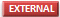Jmol: Hemoglobin

## Normal and Cooperative Binding of Dioxygen

Plots of Y (fractional saturation) vs L (pO2) are hyperbolic for Mb, but sigmoidal for Hb, suggesting cooperative binding of oxygen to Hb (binding of the first oxygen facilitates binding of second, etc).

Figure: Plots of Y (fractional saturation) vs L (pO2) are hyperbolic for Mb, but sigmoidal for Hb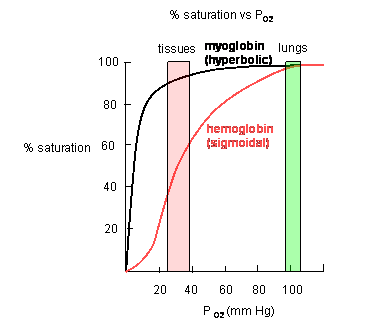In another difference, the affinity of Hb, but not of Mb, for dioxygen depends on pH. This is called the Bohr effect, after the father of Neils Bohr, who discovered it.

Figure: Bohr effect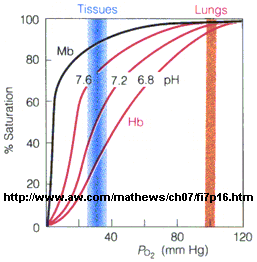Protons (decreasing pH), carbon dioxide, and bisphosphoglycerate, all allosteric ligands which bind distal to the oxygen binding sites on the heme, shift the binding curves of Hb for oxygen to the right, lowering the apparent affinity of Hb for oxygen. The same effects do not occur for Mb. These ligands regulate the binding of dioxygen to Hb.

From these clues we wish to understand the molecular and mathematical bases for the sigmoidal binding curves, and binding that can be so exquisitely regulated by allosteric ligands. The two obvious features that differ between Mb and Hb are the tetrameric nature of Hb and its multiple (4) binding sites for oxygen. Regulation of dioxygen binding is associated with conformational changes in Hb.

Based on crystallographic structures, two main conformational states appear to exists for Hb, the deoxy (or T - taut) state, and the oxy (or R -relaxed) state. The major shift in conformation occurs at the alpha-beta interface, where contacts with helices C and G and the FG corner are shifted on oxygenation.

Figure: Conformation Changes on Oxygen Binding to Deoxy-Hemoglobin (files aligned with DeepView, displayed with Pymol)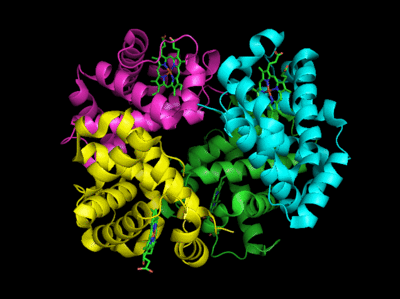The deoxy or T form is stablized by 8 salt bridges which are broken in the transition to the oxy or R state.

Figure: Salt Bridges in Deoxy Hb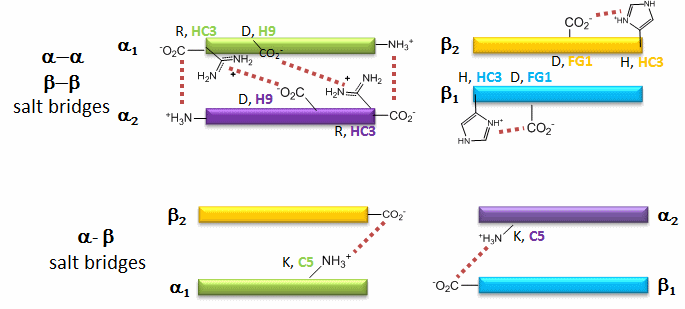Flash Movie: Salt Bridges in DeoxyHb

6 of the salt bridges are between different subunits (as expected from the above analysis), with 4 of those involving the C- or N- terminus.

In addition, crucial H-bonds between Tyr 140 (alpha chain) or 145 (on the beta chain) and the carbonyl O of Val 93 (alpha chain) or 98 (beta chain) are broken. Crystal structures of oxy and deoxy Hb show that the major conformational shift occurs at the interface between the α and βsubunits. When the heme Fe binds oxygen it is pulled into the plan of the heme ring, a shift of about 0.2 nm. This small shift leads to larger conformational changes since the subunits are packed so tightly that compensatory changes in their arrangement must occur. The proximal His (coordinated to the Fe) is pulled toward the heme, which causes the F helix to shift, causing a change in the FG corner (the sequence separating the F and G helices) at the alpha-beta interface as well as the C and G helices at the interface, which all slide past each other to the oxy-or R conformation.

Decreasing pH shifts the oxygen binding curves to the right (to decreased oxygen affinity). Increased [proton] will cause protonation of basic side chains. In the pH range for the Bohr effect, the mostly likely side chain to get protonated is His (pKa around 6), which then becomes charged. The mostly likely candidate for protonation is His 146 (on the βchain - HC3) which can then form a salt bridge with Asp 94 of the β(FG1) chain. This salt bridge stabilizes the positive charge on the His and raises its pKa compared to the oxyHb state. Carbon dioxide binds covalently to the N terminus to form a negatively charge carbamate which forms a salt bridge with Arg 141 on the alpha chain. BPG, a strongly negatively charged ligand, binds in a pocket lined with Lys 82, His 2, and His 143 (all on the beta chain). It fits into a cavity present between the β subunits of the Hb tetramer in the T state. Notice all these allosteric effectors lead to the formation of more salt bridges which stabilize the T or deoxy state. The central cavity where BPG binds between the β subunits become much smaller on oxygen binding and the shift to the oxy or R state. Hence BPG is extruded from the cavity.

The binding of H+ and CO2 helps shift the equilibrium to deoxyHb which faciliates dumping of oxygen to the tissue. It is in respiring tissues that CO2 and H+ levels are high. CO2 is produced from the oxidation of glucose through glycolysis and the Krebs cycle. In addition, high levels of CO2 increase H+ levels through the following equilibrium:

H2O + CO2 ↔ H2CO3 ↔ H+ + HCO3-

In addition, H+ increases due to production of weak acids such as pyruvic acid in glycolysis .

Hb, by binding CO2 and H+, in addition to O2, serves an additional function: it removes excess CO2 and H+ from the tissues where they build up. When deoxyHb with bound H+ and CO2 reaches the lungs, they leave as O2 builds and deoxyHb is converted to oxyHb.

### Mathematical Analysis of Cooperative Binding - The Hill Model

There are three majors models that can account for the sigmoidal binding. We will discuss all three but apply mathematical analyses to just two (Hill and MWC models).

Previously we have shown that the binding of oxygen to Mb, which can be described by the equilibrium M + L ↔ ML, and can be described mathematically by the equation for fractional saturation, Y:

$Y = \dfrac{L}{K_D + L}$

This is the equation of a hyperbola.

How does the sigmoidal binding curve for Hb arise. At least three models (Hill, MWC, and KNF) can be developed that give rise to sigmoidal binding curves. Remember, sigmoidal curves imply cooperative binding of oxygen to Hb: As oxygen binds, the next oxygen seems to bind with higher affinity (lower Kd)

Hill Model: In this model, we base our mathematical analysis on the fact that the stoichiometry of binding is not one to one, but rather 4 to 1: Perhaps a more useful equation to express the equilibrium would be M + 4L <=> ML4. For this equilibrium, we can derive an equation analogous to the equation 1 above. This equation is:

$Y = \dfrac{L^4}{K_D + L^4}$

For any given L and Kd, a corresponding Y can be calculated. Using this equation, the plot of Y vs L is not hyperbolic but sigmoidal (see next link below). Hence we're getting closer to modeling that actual data. However, there is one problem. This sigmoidal curve does not give a great fit to the actual oxygen binding curve for Hb. Maybe a better fit can be achieved by altering the exponents in equation 2. A more general equation for binding might be M + nL <=> MLn, which gives the following equation:

$Y = \dfrac{L^n}{K_D + L^n}$

If n is set to 2.8, the theoretical curve of Y vs L gives the best but still not perfect fit to the experimental data. It must seem arbitrary to change the exponent which seems to reflect the stoichiometry of binding. What molecular interpretation could you give to 2.8?

Consider another meaning of the equilibrium described above: M + 4L <=> ML4.

One interpretation of this is that all 4 oxygens bind at once to Hb. Or, alternatively, the first one binds with some low affinity, which through associated conformational changes changes the remaining 3 sites to very high affinity sites which immediately bind oxygen if the oxygen concentration is high enough. This model implies what is described as infinitely cooperative binding of oxygen.

(Notice that this equation becomes: Y = L/[Kd + L], when n =1 (as in the case with myoglobin, and in any equilibrium expression of the form M+L↔ML. Remember plots of ML vs L or Y vs L gives hyperbolas, with KD = L at Y = 0.5.)

Does KD = L at Y = 0.5? The oxygen concentration at which Y = 0.5 is defined as P50. We can substitute this value into equation 3 which gives an operational definition of KD in terms of P50.

\begin{aligned} \mathrm{Y}=0.5=\frac{\mathrm{P}_{50}^{\mathrm{n}}}{\mathrm{K}_{\mathrm{D}}+\mathrm{P}_{50}^{\mathrm{n}}} & \text { multiply both sides by } 2 \\ 1=& \frac{2 \mathrm{P}_{50}^{\mathrm{n}}}{\mathrm{K}_{\mathrm{D}}+\mathrm{P}_{50}^{\mathrm{n}}} \\ \mathrm{K}_{\mathrm{D}}+\mathrm{P}_{50}^{\mathrm{n}}=2 \mathrm{P}_{50}^{\mathrm{n}} \end{aligned}

$\mathrm{K}_{\mathrm{D}}=\mathrm{P}_{50}^{\mathrm{n}}$

Note that for this equation, KD is not the ligand concentration at half-saturation as we saw in the case with hyperbolic binding curves.

Use the sliders in the interactive graph below to explore the effect of changes in KD and n on fractional saturation.

## Mathematical Analysis of Cooperative Binding - MWC Symmetry Model

In the model MWC (Monod, Wyman, and Changeux) model, in the absence of ligand (oxygen), Hb exists in two distinct conformations, the T state (equivalent to the crystal structure of deoxyHb) and the R state (equivalent to the crystal structure of oxyHb without the oxygen). In the absence of dioxygen, the T state (T0) is greatly favored over the unliganded R state (R0) at equilibrium. An equilibrium constant (more equivalent to a dissociation constant) can be defined as L = T0/R0. (Note: L is not the ligand concentration.) In addition, let us assume that Hb can not exist with some of the monomers in a tetramer in the T state while others in the same tetramer are in the R state. Hence this model is often called the symmetry model. Finally lets assume that each oxygen can bind to either the T or R state with the dissociation constants KT and KR respectively. These constants do not depend on the number of oxygens already bound to the tetramer. Hence KR = [R0][O2]/[R1] = [R1][O2]/[R2] = ... etc., where the subscript on R refers to the number of oxygens bound to that form of R.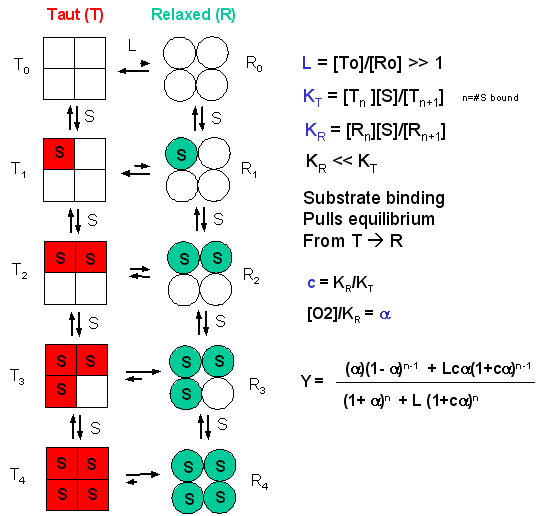Now define two new parameters:
α = [pO2]/KR which is really a normalized ligand concentration describing how many times the KR the ligand concentration is.

c = KR/KT.

If oxygen binds preferentially to the R form of Hb, c would be a small fractional number. In the limiting case, when oxygen didn't bind to the T form, KT would be infinite, and c would equal 0.

Using these definitions and equations, the following equation showing Y, fractional saturation vs α can be derived, with n, the number of binding sites per molecule, = 4 for Hb.

Y = [α (1+α)n-1 + Lcα(1+cα)n-1]/[(1+α)n +L(1+cα)n]

Figure: Y Vs alpha with varying L and c - MWC MODEL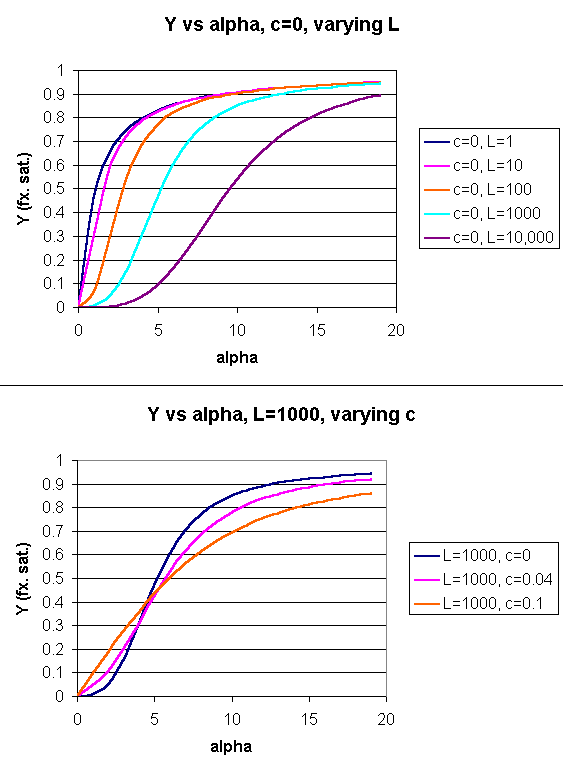When L is set at 9000 and c = 0.014, the Y vs α curve fits the experimental oxygen binding data well. The graph below shows the best experimental dioxygen binding data that I could find (obtained from a graph, not from a table), and the best fit Y vs L using a Hill coefficient of n=2.8 (fitting equation 3 above), and the best fit of Y vs L using the MWC model, with L=9000, c=0.014, and Kr = 2.8 torr.

Figure: Hb binding curves: Experimental, vs Theoretical Hill and MWC Equations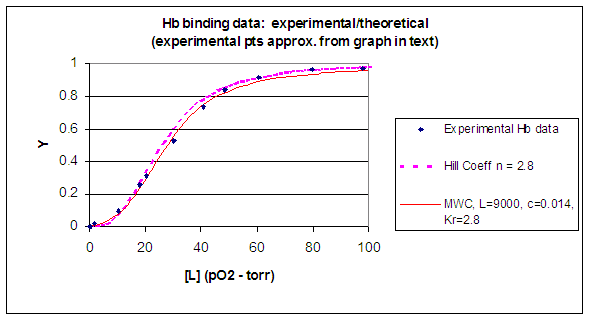Use the sliders in the interactive graph below to explore the effect of changes in L and c on fractional saturation.

Another way to think about the MWC Model

The MWC model assumes that oxygen binds to either the T or R form of Hb in a noncooperative fashion. That is KT and KR are constant, independent of the number of oxygens bound to that form. If that is so, what is the basis of the cooperative oxygen binding curves? The answer can be seen below. The red curve might reflect the binding of a ligand to the T form of a macromolecule, with Kd = 50 uM, for example. Notice that the binding curve is hyperbolic. Likewise, the blue curve might reflect the binding of a ligand to the R form of the macromolecule with Kd = 10 uM. If the T and R form are linked through the T <==> R equilibrium, this equilibrium will be shifted to the tighter binding R form with increasing ligand concentration, assuming that the ligand binds preferentially to the R form. This shifts the actual binding curve from that resembling the T form at low ligand (red) to that of the R form as ligand increases (blue), imparting sigmoidal characteristics to the actual binding curve (purple).